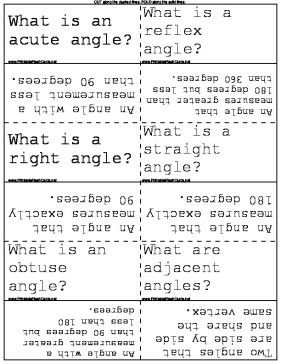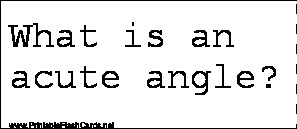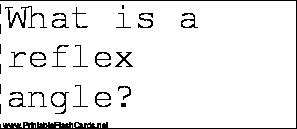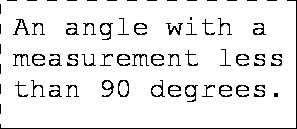Sample flash cards in this set:

Front:Back:This set includes the following cards:
What is an acute angle?An angle with a measurement less than 90 degrees.
What is a reflex angle?An angle that measures greater than 180 degrees but less than 360 degrees.
What is a right angle?An angle that measures exactly 90 degrees.
What is a straight angle?An angle that measures exactly 180 degrees.
What is an obtuse angle?An angle with a measurement greater than 90 degrees but less than 180 degrees.
What are adjacent angles?Two angles that are side by side and share the same vertex.
What are complementary angles?Two angles whose measurements add up to 90 degrees.
What are Supplementary angles?Two angles whose measurements add up to 180 degrees.
What are Corresponding Angles? What letter do they create>Two angles that are equal on parallel lines. They create an F.
What are Co-Interior Angles? What letter do they create?Two angles on parallel lines that add up to 180 degrees. They form the letter C.
What are alternate angles? What letter do they form?Two angles on parallel lines that are equal in measurement. They form the letter Z.
In converting big measurements to small measurements, what action do you take?Multiply
In converting small measurements to big measurements, what action do you take?Divide
What is the basic unit for the metric system?The Metre
How many metres are in a kilometre?1000
How many centimetres in a metre?100
How many millimetres in a centimetres?10
What is the perimeter?It is the sum of the lengths of all sides of a figure.
What are the 12 months of the year?January, February, March, April, May, June, July, August, September, October, November and December
Which months have 31 days?January, March, May, July, August and December
Which months have only 30 days?April, June, September and November
How many days in the month of February? How many during a Leap Year? How often is there a Leap Year?28 days 29 days Every 4 years
What are the four Australian seasons?Summer, Autumn, Winter and Spring
What months make up Summer?December, January and February
What months make up Autumn?March, April and May
What months make up Winter?June, July and August
What months make up Spring?September, October and November
How many hours in one day?24
How many seconds in one minute?60
How many minutes in one hour?60